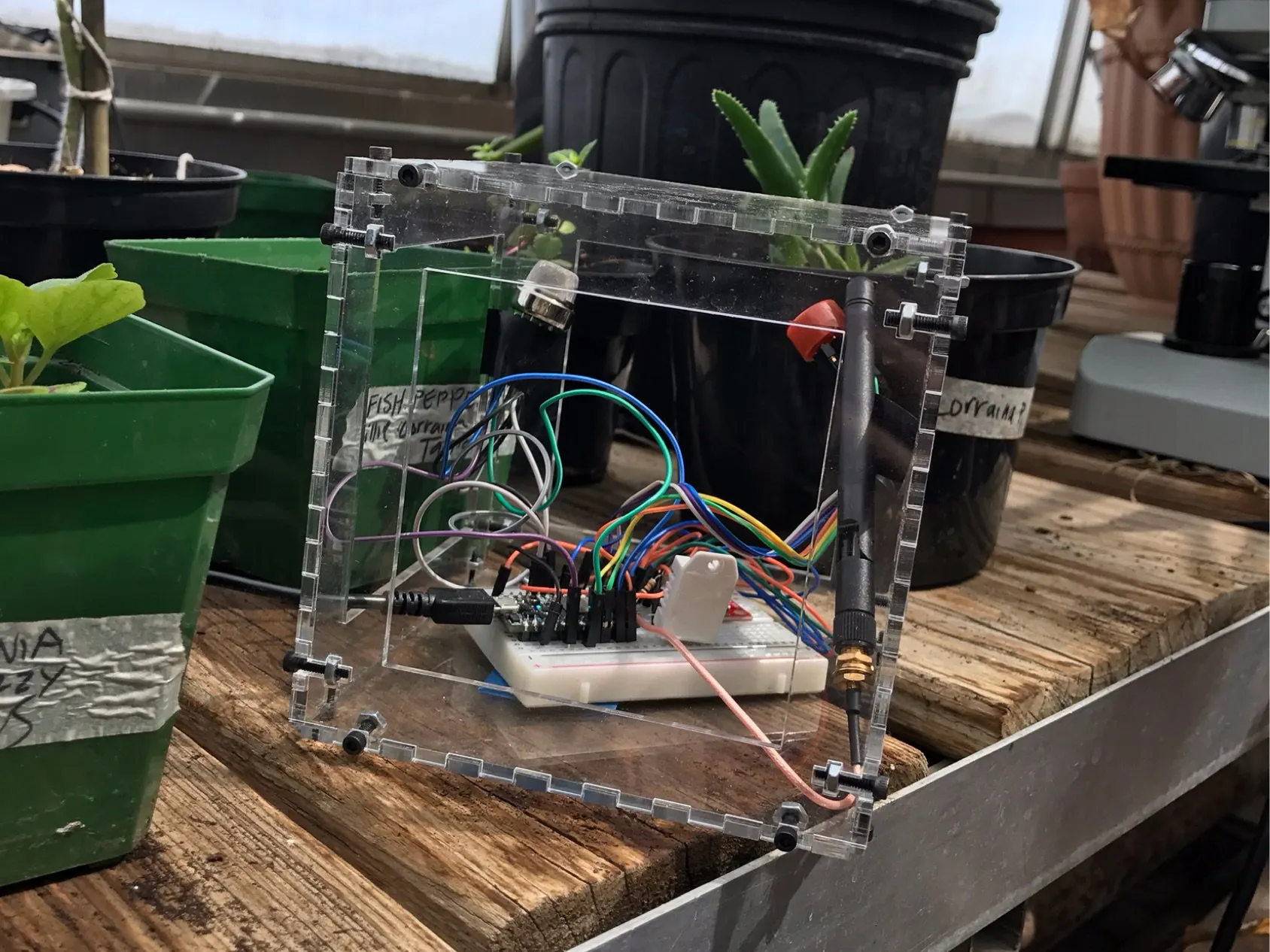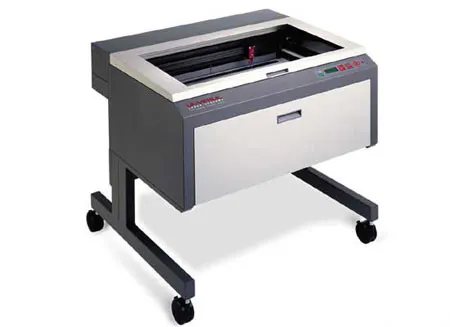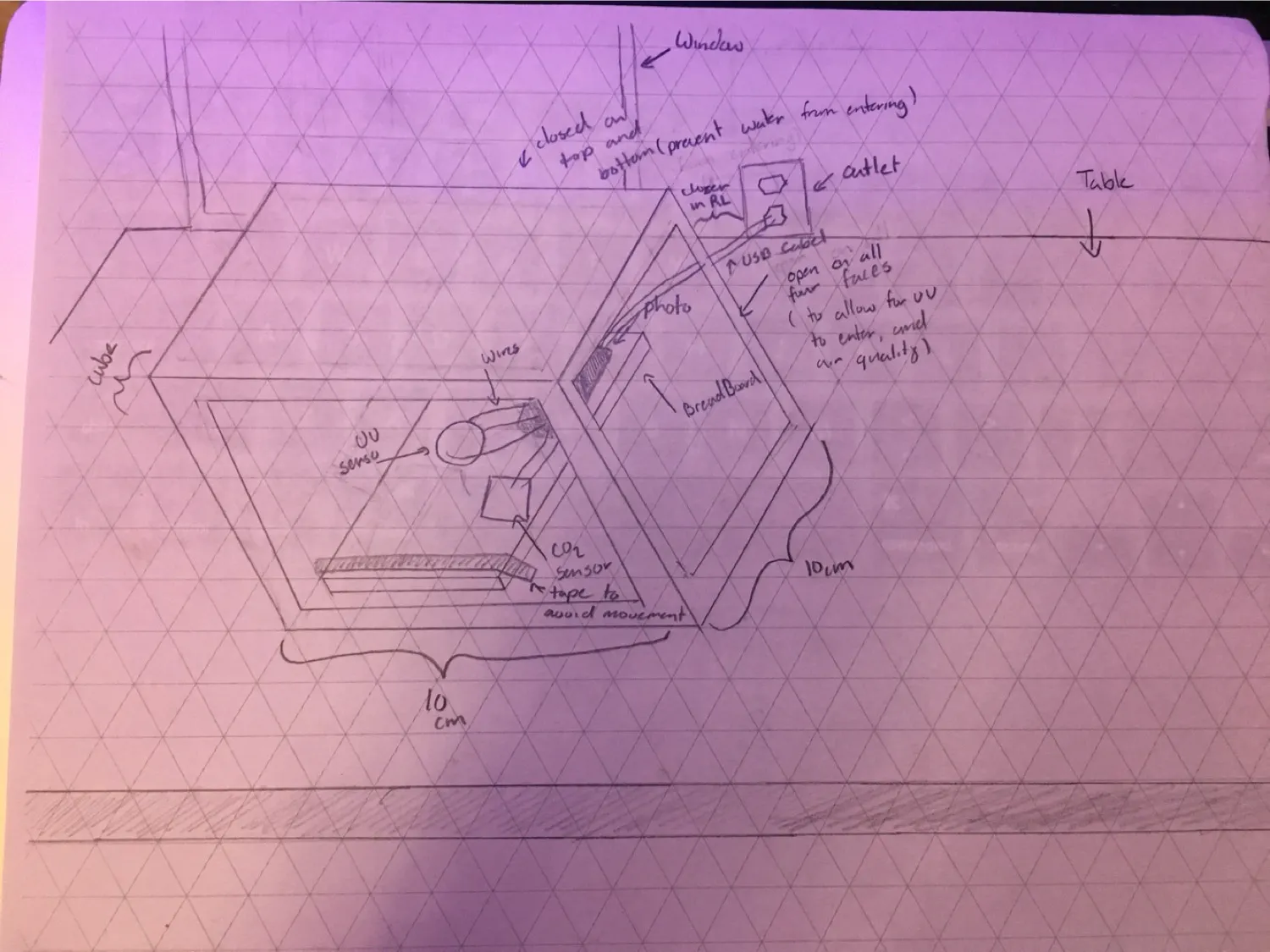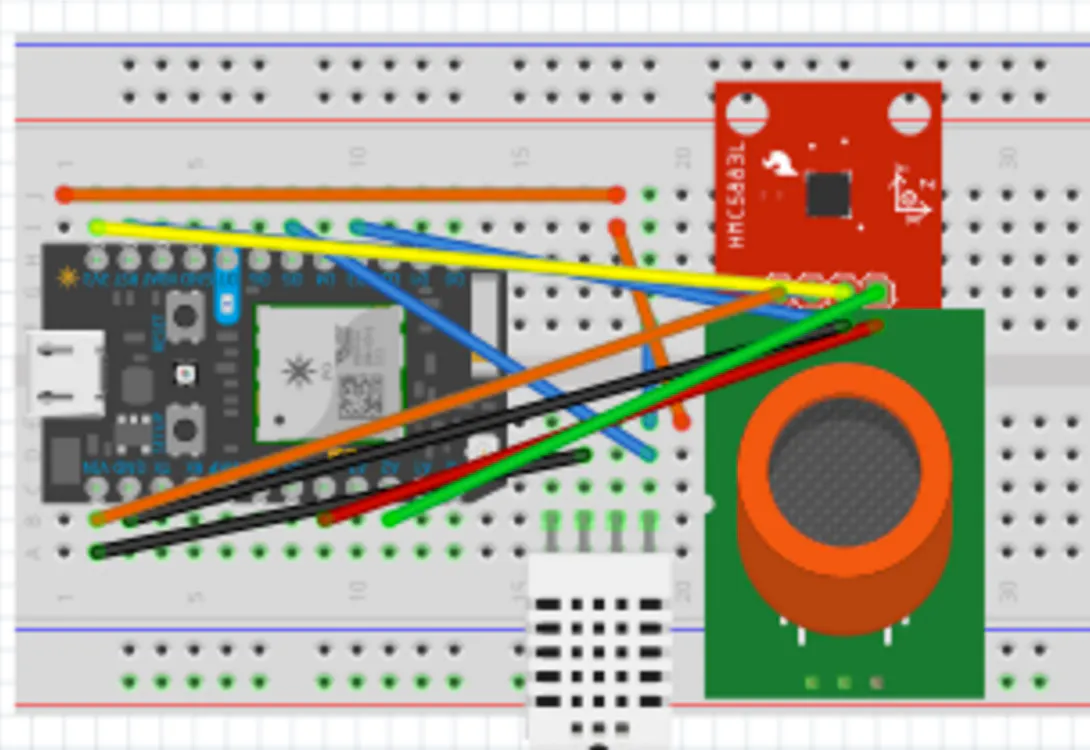# Lane of Things

Measuring UV level, Carbon Monoxide, and Hydrogen in the greenhouse of Lane Tech.

IntermediateFull instructions provided4 hours275## Things used in this project

### Hardware componentsParticle Photon
×1×1Jumper wires (generic)
×1
 SparkFun carbon monoxide sensor
×1
 SparkFun hydrogen gas sensor
×1
 SparkFun uv sensor
×1
×1
 Acrylic (generic)
×1

### Hand tools and fabrication machinesLaser cutter (generic)

## Custom parts and enclosures

### Enclosure

This is the plans for our enclosure in Adobe Illustrator. These can be cut out of acrylic on a laser cutter.

### Enclosure Diagram## Schematics

### Greenhouse## Code

### Hydrogen Sensor Code

Arduino
Used to sense levels of hydrogen in the surrounding area.
```//Takes an average of readings on a given pin
//Returns the average

int sensorValue;

void setup()
{
Serial.begin(9600);      // sets the serial port to 9600
}

void loop()
{
Serial.println(sensorValue, DEC);  // prints the value read
delay(1000);                        // wait 100ms for next reading
}

//The Arduino Map function but for floats
//From: http://forum.arduino.cc/index.php?topic=3922.0
float mapfloat(float x, float in_min, float in_max, float out_min, float out_max)
{
return (x - in_min) * (out_max - out_min) / (in_max - in_min) + out_min;
}
```

### Carbon Monoxide Sensor Code

Arduino
Used to sense Carbon Monoxide levels in the surrounding area.
```//Takes an average of readings on a given pin
//Returns the average

int sensorValue;

void setup()
{
Serial.begin(9600);      // sets the serial port to 9600
}

void loop()
{
Serial.println(sensorValue, DEC);  // prints the value read
delay(1000);                        // wait 100ms for next reading
}

//The Arduino Map function but for floats
//From: http://forum.arduino.cc/index.php?topic=3922.0
float mapfloat(float x, float in_min, float in_max, float out_min, float out_max)
{
return (x - in_min) * (out_max - out_min) / (in_max - in_min) + out_min;
}
```

### UV sensor code

Arduino
Used to sense ultraviolet wavelength levels in the surrounding area.
```int UVOUT = A0; //Output from the sensor
int REF_3V3 = A1; //3.3V power on the Arduino board

void setup()
{
Serial.begin(9600);

pinMode(UVOUT, INPUT);
pinMode(REF_3V3, INPUT);

Serial.println("ML8511 example");
}

void loop()
{

//Use the 3.3V power pin as a reference to get a very accurate output value from sensor
float outputVoltage = 3.3 / refLevel * uvLevel;

float uvIntensity = mapfloat(outputVoltage, 0.99, 2.8, 0.0, 15.0); //Convert the voltage to a UV intensity level

Serial.print("output: ");
Serial.print(refLevel);

Serial.print("ML8511 output: ");
Serial.print(uvLevel);

Serial.print(" / ML8511 voltage: ");
Serial.print(outputVoltage);

Serial.print(" / UV Intensity (mW/cm^2): ");
Serial.print(uvIntensity);

Serial.println();

delay(100);
}

//Takes an average of readings on a given pin
//Returns the average
{
unsigned int runningValue = 0;

for(int x = 0 ; x < numberOfReadings ; x++)

return(runningValue);
}

//The Arduino Map function but for floats
//From: http://forum.arduino.cc/index.php?topic=3922.0
float mapfloat(float x, float in_min, float in_max, float out_min, float out_max)
{
return (x - in_min) * (out_max - out_min) / (in_max - in_min) + out_min;
}
```

### Greenhouse Measurement code

Arduino
```// This #include statement was automatically added by the Particle IDE.

//bool WiFi.antennaSelect(AUTO | INTERNAL | EXTERNAL);

//Takes an average of readings on a given pin
//Returns the average
//Output from the sensor
int REF_3V3 = A1; //3.3V power on the Arduino board
#define DHTPIN 4            // what pin we're connected to
#define DHTTYPE DHT22
//  DHT 22 (AM2302);

DHT dht(DHTPIN, DHTTYPE);

double hum;                 // current hum
double temp;
int sensorValueC;
int sensorValueH;
int UVOUT = A0;
void setup()
{
Serial.begin(9600);

pinMode(UVOUT, INPUT);
pinMode(REF_3V3, INPUT);

Serial.println("ML8511 example");
pinMode(DHTPIN, INPUT);

Particle.variable("hum", hum);
Particle.variable("temp", temp);
Particle.variable("sensorValueC", sensorValueC);
Particle.variable("sensorValueH", sensorValueH);
Particle.variable("UVOUT", UVOUT);
}

void loop() {

double checkHum = dht.getHumidity();
double checkTemp = dht.getTempFarenheit();

if (checkHum > 0 && checkHum < 100)
hum = checkHum;

if (checkTemp > 32 && checkTemp < 100)
temp = checkTemp;

Serial.println("Temp: " + String(checkTemp));
Serial.println("Hum: " + String(checkHum));

delay(3000);

Serial.println(sensorValueC, DEC);  // prints the value read
delay(1000);
Serial.println(sensorValueH, DEC);  // prints the value read
delay(1000);
float outputVoltage = 3.3 / refLevel * uvLevel;

float uvIntensity = mapfloat(outputVoltage, 0.99, 2.8, 0.0, 15.0); //Convert the voltage to a UV intensity level

Serial.print("output: ");
Serial.print(refLevel);

Serial.print("ML8511 output: ");
Serial.print(uvLevel);

Serial.print(" / UV Intensity (mW/cm^2): ");
Serial.print(uvIntensity);

Serial.println();

delay(1000);
}

{
unsigned int runningValue = 0;

for(int x = 0 ; x < numberOfReadings ; x++)

return(runningValue);
}

//The Arduino Map function but for floats
//From: http://forum.arduino.cc/index.php?topic=3922.0
float mapfloat(float x, float in_min, float in_max, float out_min, float out_max)
{
return (x - in_min) * (out_max - out_min) / (in_max - in_min) + out_min;
}

```

## Credits

### Michael Ulie

1 project • 0 followers
LT student